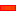• Join one Part in the Dyad to the orbiting Gear with a Pin-Joint
 • Join the other Part in the Dyad to the Base-Part with a Slide-Joint.

This Part is the 'Sliding-Part'. We do not know the motion of this Part unless we measure its motion.

 • Join the two Parts together with a Pin-Joint

####Quick Reminder of Dyads

There are five possible Dyads in Planar Kinematics: RRR, RRP, RPR, RPP and PRP.

 • The R stands for Revolute. This is the same as a Pin-Joint
 • The P stands for Prismatic. This is the same as a Slide-Joint.

 • Each Part adds three degrees-of-freedom.
 • Each Joint removes two degrees-of-freedom.

####Preparation 2B: 'Add an RRP Dyad'STEP 2: Add a Pin-Joint between the end of one of the Added-Parts and the Point with the Trace-Point on the small gear.

This is the first R in the RRP Dyad.STEP 3: Add a Slide-Joint between the Horizontal Line in the Base-Part and the Line along the centre of the other Added-Part.

This is the P in the RRP Dyad.STEP 4: Add a Pin-Joint between the Points at the end of the CAD-Lines in the Parts

This is the second R in the RRP Dyad.The Parts become Green again. The two Parts are kinematically a new Dyad.

 STEP 5: Cycle the Mechanism to make sure the Parts can complete the cycle.
 STEP 6: Edit the length of the 'Connecting Rod'Edit the length until it is approximately equal to the radius of the Trace-Pointthat the Gear-Pair generates.

When Length of Partis the same radius as the Trace-Point, the sliding-partwill approximately dwell at one end of the slide movement.

####Preparation 2B: 'Add the Rocker'In this exercise, we will not design the tool. We will simply add the Rocker to the sliding-part.

 STEP 1: Edit the sliding-part
 STEP 2: Add a vertical Line

See the image to the left.STEP 4: Add a Pin-Joint – select the Point at the end of the vertical Line and the Origin of the Part.STEP 5: Add a Motion-Dimension FB to make the Part a 'Rocker'

The angle of the Rocker relative to the vertical Line is 135º.

Name this Part the Cam-Follower.

We now want to synchronize the Motion of the Rocker with the Motion of the Slider.We want a Stationary Cam to rotate the Cam-Follower.

Add a Cam-Follower Roller sketch and Profile.

 STEP 6: Edit the Rocker / Cam-Follower.
 STEP 7: Add a Line and Circle, as indicate to the left.
 STEP 8: Close the Part-Editor
 STEP 9: Add a Profile. Use the Circle.

We can rename the Circle Profile a Cam-Follower Roller.

Tutorial and Reference Help Files for MechDesigner and MotionDesigner 13.2 + © Machine, Mechanism, Motion and Cam Design Software by PSMotion Ltd